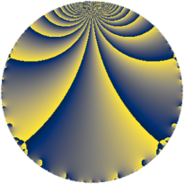Properties

 Label 8280.2.a.bcLevel $8280$ Weight $2$ Character orbit 8280.a Self dual yes Analytic conductor $66.116$ Analytic rank $0$ Dimension $2$ CM no Inner twists $1$

Related objects

Newspace parameters

 Level: $$N$$ $$=$$ $$8280 = 2^{3} \cdot 3^{2} \cdot 5 \cdot 23$$ Weight: $$k$$ $$=$$ $$2$$ Character orbit: $$[\chi]$$ $$=$$ 8280.a (trivial)

Newform invariants

 Self dual: yes Analytic conductor: $$66.1161328736$$ Analytic rank: $$0$$ Dimension: $$2$$ Coefficient field: $$\Q(\sqrt{11})$$ Defining polynomial: $$x^{2} - 11$$ Coefficient ring: $$\Z[a_1, \ldots, a_{11}]$$ Coefficient ring index: $$1$$ Twist minimal: no (minimal twist has level 2760) Fricke sign: $$-1$$ Sato-Tate group: $\mathrm{SU}(2)$

$q$-expansion

Coefficients of the $$q$$-expansion are expressed in terms of $$\beta = \sqrt{11}$$. We also show the integral $$q$$-expansion of the trace form.

 $$f(q)$$ $$=$$ $$q + q^{5} -3 q^{7} +O(q^{10})$$ $$q + q^{5} -3 q^{7} + ( 1 + \beta ) q^{11} + ( 1 - \beta ) q^{13} + \beta q^{17} + ( -5 + \beta ) q^{19} - q^{23} + q^{25} + ( 4 - \beta ) q^{29} + ( -3 - 2 \beta ) q^{31} -3 q^{35} + ( 5 + 2 \beta ) q^{37} -\beta q^{41} + ( -4 + 2 \beta ) q^{43} + ( -1 - 3 \beta ) q^{47} + 2 q^{49} + 3 \beta q^{53} + ( 1 + \beta ) q^{55} + ( 2 + 3 \beta ) q^{59} + ( 7 - \beta ) q^{61} + ( 1 - \beta ) q^{65} + ( 9 - 2 \beta ) q^{67} + ( -2 + 3 \beta ) q^{71} + ( 5 - \beta ) q^{73} + ( -3 - 3 \beta ) q^{77} + ( -2 - \beta ) q^{83} + \beta q^{85} + ( -2 - 2 \beta ) q^{89} + ( -3 + 3 \beta ) q^{91} + ( -5 + \beta ) q^{95} + 12 q^{97} +O(q^{100})$$ $$\operatorname{Tr}(f)(q)$$ $$=$$ $$2 q + 2 q^{5} - 6 q^{7} + O(q^{10})$$ $$2 q + 2 q^{5} - 6 q^{7} + 2 q^{11} + 2 q^{13} - 10 q^{19} - 2 q^{23} + 2 q^{25} + 8 q^{29} - 6 q^{31} - 6 q^{35} + 10 q^{37} - 8 q^{43} - 2 q^{47} + 4 q^{49} + 2 q^{55} + 4 q^{59} + 14 q^{61} + 2 q^{65} + 18 q^{67} - 4 q^{71} + 10 q^{73} - 6 q^{77} - 4 q^{83} - 4 q^{89} - 6 q^{91} - 10 q^{95} + 24 q^{97} + O(q^{100})$$

Embeddings

For each embedding $$\iota_m$$ of the coefficient field, the values $$\iota_m(a_n)$$ are shown below.

For more information on an embedded modular form you can click on its label.

Label $$\iota_m(\nu)$$ $$a_{2}$$ $$a_{3}$$ $$a_{4}$$ $$a_{5}$$ $$a_{6}$$ $$a_{7}$$ $$a_{8}$$ $$a_{9}$$ $$a_{10}$$
1.1
 −3.31662 3.31662
0 0 0 1.00000 0 −3.00000 0 0 0
1.2 0 0 0 1.00000 0 −3.00000 0 0 0
 $$n$$: e.g. 2-40 or 990-1000 Significant digits: Format: Complex embeddings Normalized embeddings Satake parameters Satake angles

Atkin-Lehner signs

$$p$$ Sign
$$2$$ $$-1$$
$$3$$ $$-1$$
$$5$$ $$-1$$
$$23$$ $$1$$

Inner twists

This newform does not admit any (nontrivial) inner twists.

Twists

By twisting character orbit
Char Parity Ord Mult Type Twist Min Dim
1.a even 1 1 trivial 8280.2.a.bc 2
3.b odd 2 1 2760.2.a.l 2
12.b even 2 1 5520.2.a.bo 2

By twisted newform orbit
Twist Min Dim Char Parity Ord Mult Type
2760.2.a.l 2 3.b odd 2 1
5520.2.a.bo 2 12.b even 2 1
8280.2.a.bc 2 1.a even 1 1 trivial

Hecke kernels

This newform subspace can be constructed as the intersection of the kernels of the following linear operators acting on $$S_{2}^{\mathrm{new}}(\Gamma_0(8280))$$:

 $$T_{7} + 3$$ $$T_{11}^{2} - 2 T_{11} - 10$$ $$T_{13}^{2} - 2 T_{13} - 10$$ $$T_{17}^{2} - 11$$

Hecke characteristic polynomials

$p$ $F_p(T)$
$2$ $$T^{2}$$
$3$ $$T^{2}$$
$5$ $$( -1 + T )^{2}$$
$7$ $$( 3 + T )^{2}$$
$11$ $$-10 - 2 T + T^{2}$$
$13$ $$-10 - 2 T + T^{2}$$
$17$ $$-11 + T^{2}$$
$19$ $$14 + 10 T + T^{2}$$
$23$ $$( 1 + T )^{2}$$
$29$ $$5 - 8 T + T^{2}$$
$31$ $$-35 + 6 T + T^{2}$$
$37$ $$-19 - 10 T + T^{2}$$
$41$ $$-11 + T^{2}$$
$43$ $$-28 + 8 T + T^{2}$$
$47$ $$-98 + 2 T + T^{2}$$
$53$ $$-99 + T^{2}$$
$59$ $$-95 - 4 T + T^{2}$$
$61$ $$38 - 14 T + T^{2}$$
$67$ $$37 - 18 T + T^{2}$$
$71$ $$-95 + 4 T + T^{2}$$
$73$ $$14 - 10 T + T^{2}$$
$79$ $$T^{2}$$
$83$ $$-7 + 4 T + T^{2}$$
$89$ $$-40 + 4 T + T^{2}$$
$97$ $$( -12 + T )^{2}$$